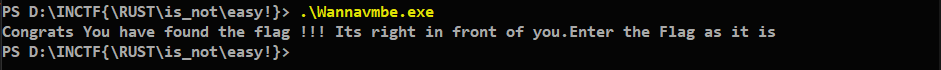# Wannavmbe - InCTF Internationals 2019

Intended solution of Wannavmbe challenge from InCTF Internationals 2019

tl;dr

• Challenge is a VM.
• Reverse Instruction types and implementation.
• Understand that it has a fucntion which takes the base64 of CWD (Current working directory).
• Find the corrcect directory where it needs to be placed.

Challenge Points: 1000
Challenge Solves: 0
Challenge Author: Freakston

## Write-up

In this challenge we are given a PE32+ executable for windows. Running the file at first looks like it does nothing.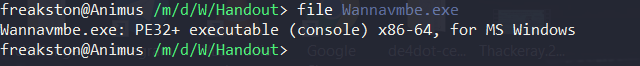Openning the file in IDA we get to a main function which does not look useful. On checking the strings out we find out that the binary was written in RUST language. Then we find the congratulatory string in it.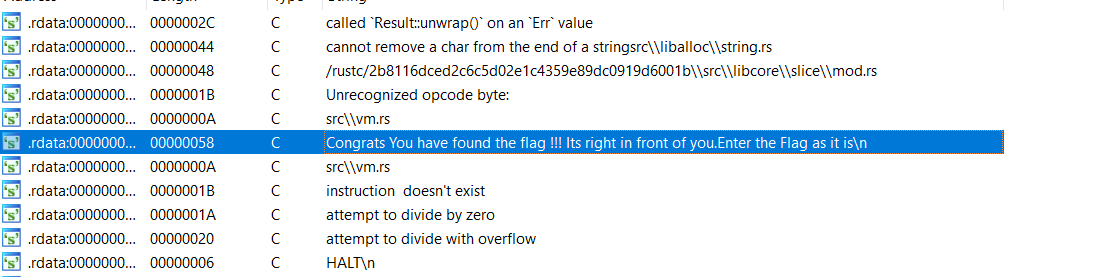On tracing it back to the function we get a huge function which looks like a VM (“The name tells us its a VM ;).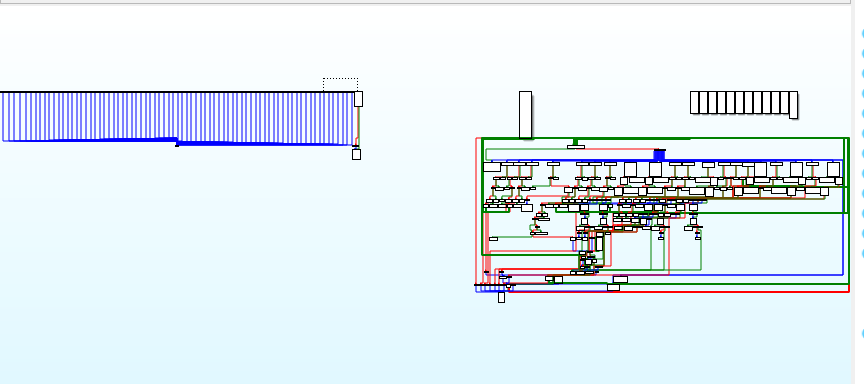On analysing this particular function we find a switch case which is repeated a lot of times with minor changes to it.

``````    case 0x17ui64:

*v1 = v3 + 1;
v82 = xmmword_140020310;
v83 = xmmword_140020320;
v84 = xmmword_140020330;
v85 = xmmword_140020340;
v86 = xmmword_140020350;
v87 = xmmword_140020360;
v88 = xmmword_140020370;
v89 = xmmword_140020380;
v90 = xmmword_140020390;
v91 = xmmword_1400203A0;
v92 = xmmword_1400203B0;
v93 = xmmword_1400203C0;
v94 = xmmword_1400203D0;
v95 = xmmword_1400203E0;
v96 = xmmword_1400203F0;
v97 = xmmword_140020400;
v98 = 73;
v106 = 0;
sub_140007D40(&v99);
if ( (_QWORD)v99 == 1i64 )
{
v102 = *(__int128 *)((char *)&v99 + 8);
sub_14001A420("called `Result::unwrap()` on an `Err` value", 43i64, &v102, &off_140020440);
}
v103 = v101;
v102 = *(__int128 *)((char *)&v99 + 8);
v106 = 0;
sub_140008810(&v80, v2);
v106 = 0;
sub_140008750(&v99, &v80);
if ( (_QWORD)v99 == 1i64 )
{
v103 = v101;
v102 = *(__int128 *)((char *)&v99 + 8);
sub_14001A420("called `Result::unwrap()` on an `Err` value", 43i64, &v102, &off_140020460);
}
v105 = v101;
v104 = *(__int128 *)((char *)&v99 + 8);
*(_QWORD *)&v99 = *((_QWORD *)&v99 + 1);
*((_QWORD *)&v99 + 1) += v101;
v100 = 3i64;
v106 = 1;
sub_140004670(v2, &v99);
if ( *((_QWORD *)&v104 + 1) )
sub_140004AE0(v104, *((__int64 *)&v104 + 1), 1i64);
v105 = v103;
v104 = v102;
v106 = 1;
sub_1400041A0(&v99, &v104);
if ( *((_QWORD *)&v104 + 1) )
sub_140004AE0(v104, *((__int64 *)&v104 + 1), 1i64);
v105 = v100;
v104 = v99;
v56 = *((int *)v1 + 14);
v106 = 1;
v57 = sub_140001130(&v104, v56);
v58 = *((int *)v1 + 19);
if ( v58 > 0x40 )
{
v106 = 1;
sub_14001A2F0(&off_140020620, v58, 65i64);
}
if ( v57 != *((_DWORD *)&v82 + v58) )
{
v106 = 1;
sub_14000C340(0x100u); \\ This is the exit function
}
``````

On further analysis we find a function that looks like Base64 . On going through the XREFS we find that this is being called in the above function. Now lets check the arguements being passed.

Now we have one task that is to extract the bytecode of the VM function.We have many methods of doing this. One would be to write a script to extract the value of each switch case or extract the whole array from the memory . If we go ahead with the first method we have one advantage of knwoing which case was the last one to be excecuted.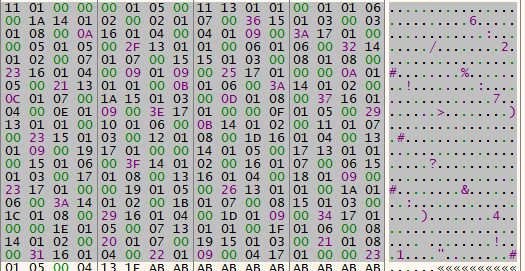Now we know that the first bytecode to be excecuted is 0x11.

Let’s have a look at the switch case.

On having a deep look at the 0x11th switch case we find a function which takes the current dir and removes the first 3 characters from it. Then it passes this string to the base64 function as one of the argumenets. Once this is done it checks if the base64 string is 36 characters long.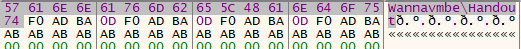Now we know what happens in this challenge. The excecutable gets the Current working directory and removes the first 3 characters and passes it to the base64 function.

Now on looking at the next few switch cases we find out that once the length check is done the VM moves onto check the base64 character by character.

The character by character check part -

``````          *v1 = v3 + 1;
v82 = xmmword_7FF65B900310;
v83 = xmmword_7FF65B900320;
v84 = xmmword_7FF65B900330;
v85 = xmmword_7FF65B900340;
v86 = xmmword_7FF65B900350;
v87 = xmmword_7FF65B900360;
v88 = xmmword_7FF65B900370;
v89 = xmmword_7FF65B900380;
v90 = xmmword_7FF65B900390;
v91 = xmmword_7FF65B9003A0;
v92 = xmmword_7FF65B9003B0;
v93 = xmmword_7FF65B9003C0;
v94 = xmmword_7FF65B9003D0;
v95 = xmmword_7FF65B9003E0;
v96 = xmmword_7FF65B9003F0;
v97 = xmmword_7FF65B900400;
v98 = 73;
v106 = 0;
Getcurdir((__int64)&v99);
if ( (_QWORD)v99 == 1i64 )
{
v102 = *(__int128 *)((char *)&v99 + 8);
sub_7FF65B8FA420(
(__int64)"called `Result::unwrap()` on an `Err` value",
43i64,
(__int64)&v102,
(__int64)&off_7FF65B900440);
}
v103 = v101;
v102 = *(__int128 *)((char *)&v99 + 8);
v106 = 0;
sub_7FF65B8E8810((__int64)&v80, (__int64)v2);
v106 = 0;
sub_7FF65B8E8750(&v99, &v80);
if ( (_QWORD)v99 == 1i64 )
{
v103 = v101;
v102 = *(__int128 *)((char *)&v99 + 8);
sub_7FF65B8FA420(
(__int64)"called `Result::unwrap()` on an `Err` value",
43i64,
(__int64)&v102,
(__int64)&off_7FF65B900460);
}
v105 = v101;
v104 = *(__int128 *)((char *)&v99 + 8);
*(_QWORD *)&v99 = *((_QWORD *)&v99 + 1);
*((_QWORD *)&v99 + 1) += v101;
v100 = 3i64;
v106 = 1;
sub_7FF65B8E4670(v2, &v99);
if ( *((_QWORD *)&v104 + 1) )
sub_7FF65B8E4AE0(v104, *((__int64 *)&v104 + 1), 1i64);
v105 = v103;
v104 = v102;
v106 = 1;
Base6((__int64)&v99, (__int64 *)&v104);
if ( *((_QWORD *)&v104 + 1) )
sub_7FF65B8E4AE0(v104, *((__int64 *)&v104 + 1), 1i64);
v105 = v100;
v104 = v99;
v72 = *((int *)v1 + 10);
v106 = 1;
v73 = sub_7FF65B8E1130(&v104, v72);
v74 = *((int *)v1 + 15);
if ( v74 > 0x40 )
{
v106 = 1;
sub_7FF65B8FA2F0(&off_7FF65B900680, v74, 65i64);
}
if ( v73 != *((_DWORD *)&v82 + v74) )
{
v106 = 1;
sub_7FF65B8EC340(5u); //The exit function
}
goto LABEL_141;

``````

The final base64 string is -

``````SU5DVEZ7XFJVU1RcaXNfbm90XGVhc3khfQ==
``````

On decrypting the base64 string we get

``````INCTF{\RUST\is_not\easy!}
``````

And there you go you have the flag :)